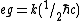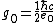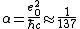# Magnetic monopoles

Also found in: Dictionary, Thesaurus.

## Magnetic monopoles

Magnetically charged particles. Such particles are predicted by various physical theories, but so far all experimental searches have failed to demonstrate their existence.

The fundamental laws governing electricity and magnetism become symmetric if particles exist that carry magnetic charge. Current understanding of electromagnetic physical phenomena is based on the existence of electric monopoles, which are sources or sinks of electric field lines (illus. a), and which when set into motion generate magnetic fields. The magnetic field lines produced by such a current have no beginning or end and form closed loops. All magnetic fields occurring in nature can be explained as arising from currents. However, theories of electromagnetism become symmetric if magnetic charges also exist. These would be sources or sinks of magnetic field and when set into motion would generate electric fields whose lines would be closed without ends (illus. b). See Electric field

In 1931 P. A. M. Dirac found a more fundamental reason for hypothesizing magnetic charges, when he showed that this would explain the observed quantization of electric charge. He showed that all electric and magnetic charges e and g must obey Eq.

(1)(1), where k must be an integer and &planck; is Planck's constant divided by 2&pgr;. Equation (1) can be satisfied only if all electric and magnetic charges are integer multiples of an elementary electric charge e0 and an elementary magnetic charge g0. Since the size of the elementary electric charge, the charge carried by an electron or proton, is known experimentally, Dirac's equation predicts the size of the elementary magnetic charge to be given by Eq. (2). Since the fine
(2)(3)structure constant α is given by Eq. (3), the elementary magnetic charge g0 is about 68.5 times larger than the elementary electric charge e0. See Fine structure (spectral lines), Fundamental constants

In 1983 a successful theoretical unification of the electromagnetic and weak forces culminated in the detection of the W+, the W-, and the Z0 particles predicted by the theory. This success has encouraged the search for a grand unification theory that would include the electroweak force and the nuclear or color force under one consistent description. In 1974 G. 't Hooft and independently A. M. Polyakov showed that magnetically charged particles are necessarily present in all true unification theories (those based on simple or semisimple compact groups). These theories predict the same long-range field and thus the same charge g0 as the Dirac solution; now, however, the near field is also specified, leading to a calculable mass. The SU(5) model predicts a monopole mass of 1016 GeV/c2, while theories based on supersymmetry or Kaluza-Klein models yield even higher masses up to the Planck mass of 1019 GeV/c2. See Electroweak interaction, Fundamental interactions, Grand unification theories, Quantum gravitation, Supergravity, Supersymmetry

There are two classes of magnetic monopole detectors, superconducting and conventional. On February 14, 1982, a prototype superconducting detector operating at Stanford University observed a single candidate event. Since then a number of groups have operated larger second- and third-generation detectors, and their combined data have placed a limit on the monopole flux more than 3000 times lower than the value from the data set that included the original event. Thus the possibility that this event was caused by the passage of a magnetic monopole has been largely discounted.

McGraw-Hill Concise Encyclopedia of Physics. © 2002 by The McGraw-Hill Companies, Inc.
References in periodicals archive ?
The central idea of the spin-ice magnetic monopoles is the existence of low energy excitations of the spin field chain when a spin flip is produced between two contiguous crystalline tetrahedral basis.
Application: Although the research is still at early stage, scientists hope isolating a magnetic monopole will lead to applications such as "magnetricity", a form of magnetic current that could be used instead of electrons in parallel computing.
Among others, in 1931, the physicist Paul Dirac was led by his calculations to the conclusion that magnetic monopoles can exist at the end of tubes - called Dirac strings - that carry magnetic field.
The accelerated growth Guth proposed didn't just dilute magnetic monopoles to unobservably low densities.
By the Grand Unified Theory (see 1973, Proton Decay), it would seem that magnetic monopoles must exist but must be so monstrously massive that there could only have been enough energy to form them in the immediate instants following the big bang.
where [m.sup.m], [n.sup.m], and [q.sup.m] are defined as the mass, total number, and charge of magnetic monopoles, respectively.
Challenged by this puzzle, scientists successfully constructed systems that effectively act as magnetic monopoles: certain crystal structures were made to behave like magnetic monopoles.
momentum density [MATHEMATICAL EXPRESSION NOT REPRODUCIBLE IN ASCII], used in (2), is independent by the assumption of existence or nonexistence of magnetic monopoles and currents, but it is an intrinsic property of the electromagnetic field , known as a consequence of charge symmetry of Maxwell equations .
Thus [I.sub.R] is always a physically measurable quantity and is present in [any.bar] physical measurement via the deltronempowered version of [[??].sub.R] (the superscript [conjunction] refers to deltron-empowerment via the coupling mechanism between electric and magnetic monopoles).
da Hora, "Generalized BPS magnetic monopoles," Physical Review D: Particles, Fields, Gravitation and Cosmology, vol.
A regular theory of magnetic monopoles and its implications.
This subtle realm of inverse spacetime is characterized by superluminal magnetic monopoles of negative mass, together with electric dipoles and subtle or "magnetoelectric" light.

Site: Follow: Share:
Open / Close